GET THE APP

Coefficient Bounds of Functions over the Quaternions

# Journal of Generalized Lie Theory and Applications

ISSN: 1736-4337

Open Access
Submit Manuscript arrow_forward arrow_forward2021 Conference Announcement - (2024) Volume 9, Issue 7

# Coefficient Bounds of Functions over the Quaternions

Olubunmi A. Fadipe-Joseph*, Olanike R. Oluwaseyi and O. E. Opaleye
*Correspondence: Olubunmi A. Fadipe-Joseph, Department of Mathematics, University of Ilorin, P.M.B. 1515, Ilorin, Nigeria, Tel: 2348033972496, Email: ,
Department of Mathematics, University of Ilorin, P.M.B. 1515, Ilorin, Nigeria

Received: 26-Sep-2022, Manuscript No. jbbs-23-87910; Editor assigned: 28-Sep-2022, Pre QC No. P-87910; Reviewed: 12-Oct-2022, QC No. Q-87910; Revised: 18-Oct-2022, Manuscript No. R-87910; Published: 26-Oct-2022 , DOI: 10.37421/1736-4337.2020.14.300 , QI Number: 1
Citation: Imbalzano, Marco. â??Making Use of Machine Learning Algorithms for Multimodal Equipment to Assist in COVID-19's Assessment.â? J Bioengineer & Biomedical Sci 12 (2022): 325.
Copyright: Â© 2022 Imbalzano M. This is an open-access article distributed under the terms of the Creative Commons Attribution License, which permits unrestricted use, distribution, and reproduction in any medium, provided the original author and source are credited.

Sources of funding : 1

## Abstract

1

#### Keywords

Quaternions • Chebyshev polynomials • Modified sigmoid function

#### Introduction

Let a complex number z be defined as z = x + yi, where x,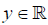and i2=-1. Let the quaternion field H be defined as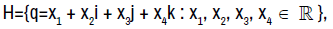where the imaginary units i,j,k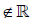satisfy

i2= j2= k2= −1, ij=−ji=k, jk=−kj=i, ki=−ik=j.

The quaternion extends the class of complex numbers. Recall that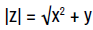Similarly,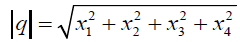We denote by B the open unit ball centered at the origin in H, i.e.,

B={q ∈ H : |q| < 1} .

Let A be the class of functions of the form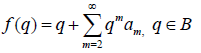that are holomorphic in the open unit ball {B=q ∈H:|q| < 1} [1-4].

The theory of functions over the complex field is very rich. These functions are very useful in the analysis of practical problems of hydrodynamics, aerodynamics, elasticity, electrodynamics and the natural sciences. The quaternions are four dimensional. Therefore, it is important to study the geometric theory for quaternionic functions.

The Chebyshev polynomials of the first kind Tn(t), t[-1, 1] have the generating function of the form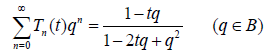and that of second kind is :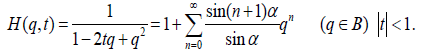Note that if t = cosα, α ∈ ( −π/3 , π/3 ) then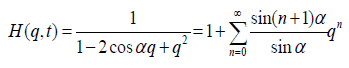Thus,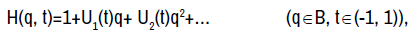where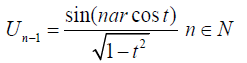are the Chebyshev polynomials of the second kind. Also,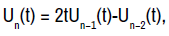so that,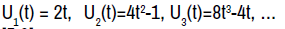(1.2) [5,6].

Lemma 1.1

If ω(q)=b1q+b2q2+b1≠0 is analytic and satisfy |ω(q)|<1 in the unit ball B, then for each 0<R<1, |ω’ (q)|<1 and ω(Reiθ)<1 unless ω(q) = eq for some real number σ

Lemma 1.2

Let ω ∈ Ω={ω ∈ A : |w(q)| ≤ |q| , q ∈ B}.

If ω ∈ Ω, ω(q) =(q ∈ B), then

|cn| ≤ 1 n = 1, 2, …. , |c2| ≤ 1 − |c1|2 (1.3)

and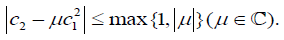(1.4)

The result is sharp. The functions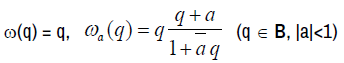are extremal functions.

#### Main Results

Definition 2.1

The modified sigmoid function is defined in series form as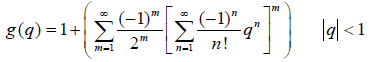Theorem 2.1

Let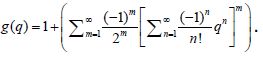Then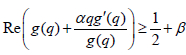for some real α ≥ 0;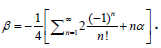Proof: By definition,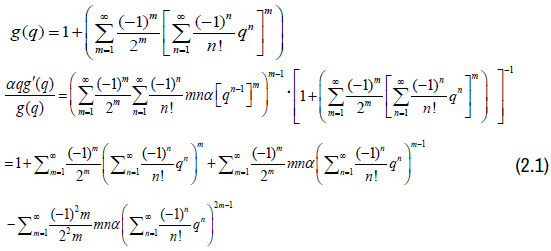When m=1 in (2.1),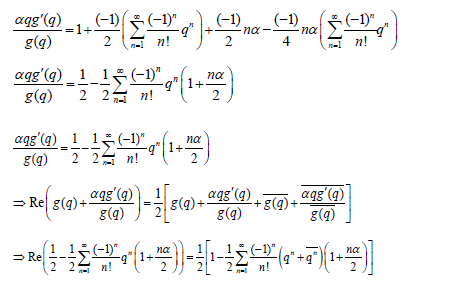Since q = Re,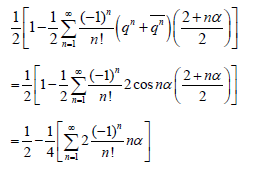Hence,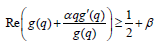Where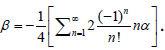Definition 2.2

A function f∈A is said to be in the class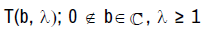if the following subordination holds.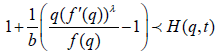Theorem 2.2

If f (q) as defined in (1.1) belongs to the class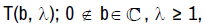then,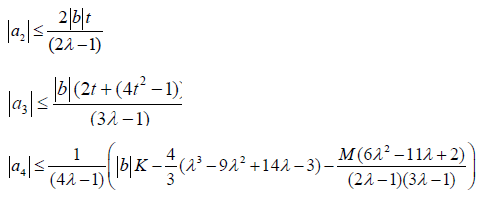where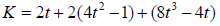and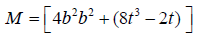Proof: Suppose f ∈ T (b, λ), then by definition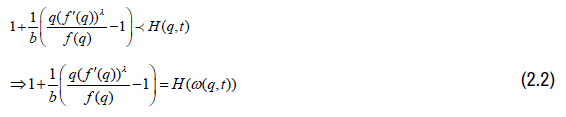Now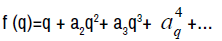and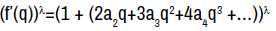Using binomial expansion,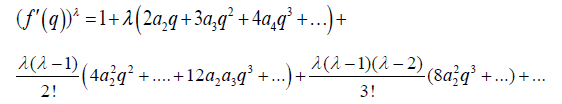This implies that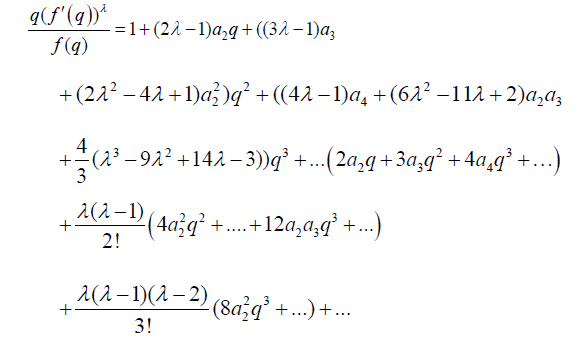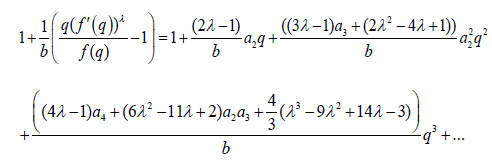(2.3)

Since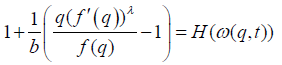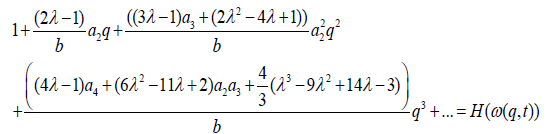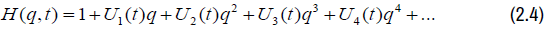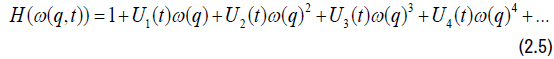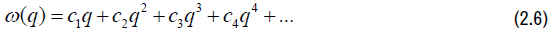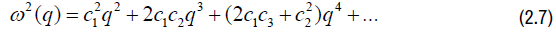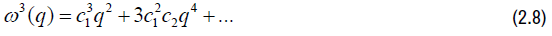substituting (2.6),(2.7) and (2.8) in (2.4) we have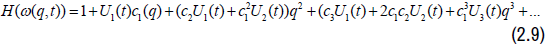Equating (2.3) and (2.9) and comparing coefficients q, q2 and q3, applying equation (1.2) and Lemma 1.2 we have the result.

2.1 Fekete-Szego˙ Inequality

The Fekete-Szego˙ functional for the class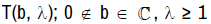is given here.

Theorem 2.3 If f (q) belongs to the class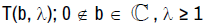then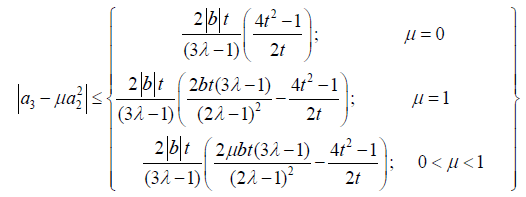#### Conclusion

The classical geometric function theory was extended to functions of quaternionic variables in this work. A new class of function involving the Chebyshev polynomial of the second kind was defined. The coefficient bounds and the Fekete-Szego˙ functional for quaternionic functions were established using subordination principle.

#### Journal Highlights

###### Citations: 2083

Journal of Generalized Lie Theory and Applications received 2083 citations as per Google Scholar report